Courses

# Test: Data Handling - 1

## 10 Questions MCQ Test Mathematics (Maths) Class 6 | Test: Data Handling - 1

Description
This mock test of Test: Data Handling - 1 for Class 6 helps you for every Class 6 entrance exam. This contains 10 Multiple Choice Questions for Class 6 Test: Data Handling - 1 (mcq) to study with solutions a complete question bank. The solved questions answers in this Test: Data Handling - 1 quiz give you a good mix of easy questions and tough questions. Class 6 students definitely take this Test: Data Handling - 1 exercise for a better result in the exam. You can find other Test: Data Handling - 1 extra questions, long questions & short questions for Class 6 on EduRev as well by searching above.
QUESTION: 1

### The following pictograph shows the number of absentees in a class of 30 students during the previous week. Which day had full attendance?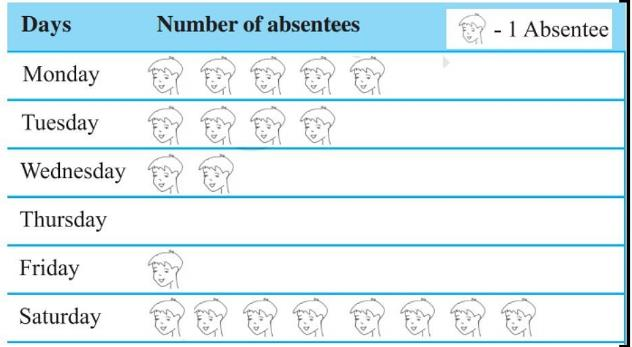Solution:

Since the day Thursday has no absentee therefore in that day all was present and had full attendance.

QUESTION: 2

### The following pictograph shows the number of absentees in a class of 30 students during the previous week. What was the total number of absentees on Tuesday?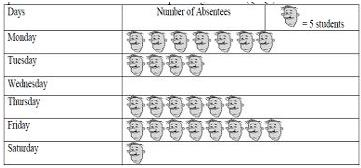Solution:

One emoji means 5 students so there are 4 emoji like these are absent on Tuesday. So, 5 multiplied by 4 = 20

QUESTION: 3

### The following pictograph shows the number of absentees in a class of 30 students during the previous week. On which day 30 students were absent?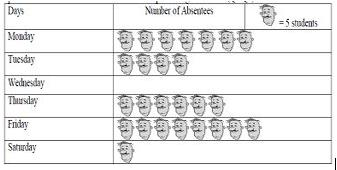Solution:

Here, one face stands for 5 students and on Thursday the pictograph shows 6 faces that mean 5 * 6  = 30

QUESTION: 4

The colours of fridges preferred by people living in a locality are shown by the following pictograph. How many people liked red colour?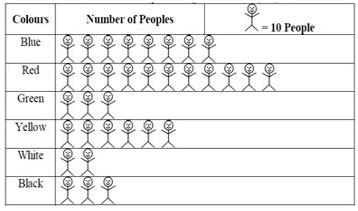Solution:

As given, 1 people  =  10
so 11 people are there.
Therefore,  11 * 10 = 110

QUESTION: 5

The following pictograph shows the number of absentees in a class of 30 students during the previous week. On which day were the maximum number of students absent?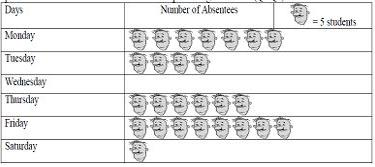Solution:

Friday is the correct ✔ answer.
As we can see, 8 *5 = 40

QUESTION: 6

The colours of fridges preferred by people living in a locality are shown by the following pictograph. Find the number of people preferring blue colour.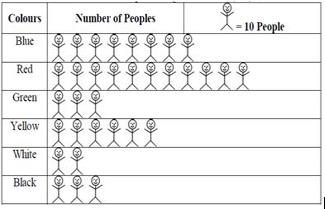Solution:

As we can see, 1 person = 10 people,
so the blue color represents 8 people.
Therefore, 8 people
=  8 * 10 ⇒ 80

QUESTION: 7

The following pictograph shows the number of absentees in a class of 30 students during the previous week. What was the total number of absentees in that week?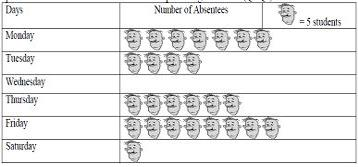Solution:

Each pic counts as 5 people. There are 26 pics.
So, 26 * 5 = 130

QUESTION: 8

The following pictograph shows the number of absentees in a class of 60 students during the previous week. On which day 5 students were absent?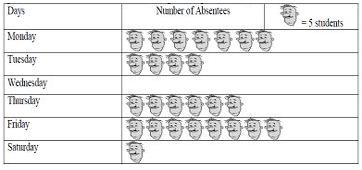Solution:

This is so because the scale shows figure of,
1 boy = 5 students

QUESTION: 9

The colours of fridges preferred by people living in a locality are shown by the following pictograph. Which colour preferred most?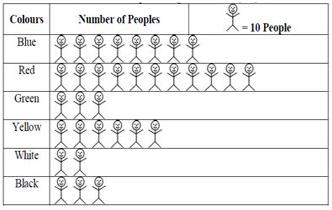Solution:

As we can see that there are larger no. of people in the red color representation.

QUESTION: 10

The colours of fridges preferred by people living in a locality are shown by the following pictograph. Which colour preferred least?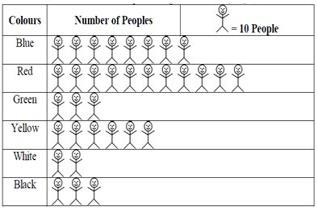Solution:

White is the correct answer.
Because only 20 people preferred the white colour.